# 基于学习的计算机视觉 （二） ：卷积神经网络

2017 年 12 月 14 日

## 神经网络的发展历史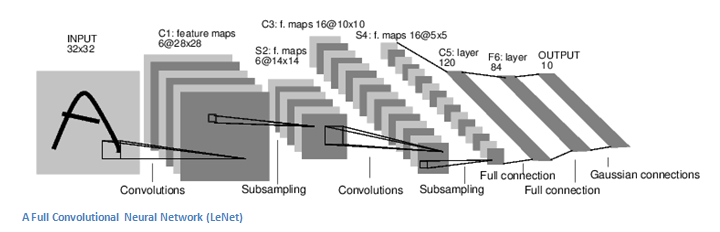• 如何提高模型的速度。提高速度对 CNN 在移动端的部署至关重要。通过去掉 max pooling，改用 stride 卷积，使用 group 卷积，定点化等方法，人脸检测、前后背景分割等 CNN 应用已经在手机上大规模部署。

## 神经网络 vs 卷积神经网络

• 参数爆炸
以 200x200x3 的图像为例，如果输入层之后的 hidden layer 有 100 个神经元，那么参数量会达到 200x200x3x100=1200 万。显然有如此多参数的模型是难以训练且容易过拟合的。
• 平移不变性
对很多图像问题，我们希望模型满足一定的平移不变性。 例如对图像分类问题，我们希望物体出现在图片的任何位置上，模型都能正确识别出物体。
• 局部相关性
在大数据等问题中，输入维度之间不存在显式的拓扑关系，因此适合使用神经网络（全连接层）进行建模。但对于计算机视觉的问题，输入图片的相邻像素之间存在天然的拓扑关系。例如，判断图片中某个位置是否有物体时，我们只需要考虑这个位置周边的像素就可以了，而不需要像传统神经网络那样将图片中所有像素的信息作为输入。

## 卷积神经网络

### 网络结构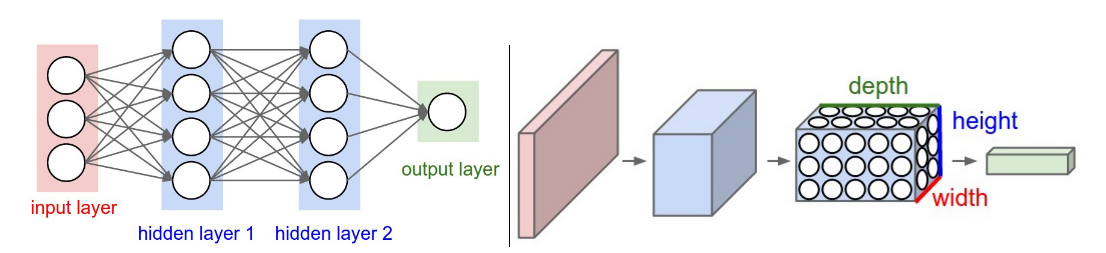### 卷积 (Convolution)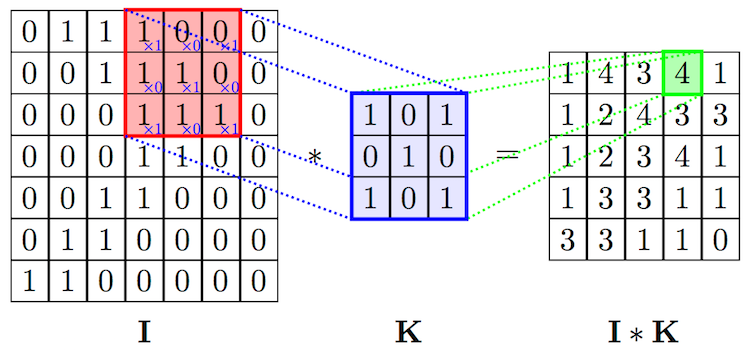$${conv}(I, K)_{xy} = \sum_{i=1}^h \sum_{j=1}^w \sum_{k=1}^d {K_{ijk} \cdot I_{x + i - 1, y + j - 1, k}} + b$$

• depth 指的是输出 channel 的数量, 对应于卷积层中 filter 的数量
• stride 指的是 filter 每次滑动的步长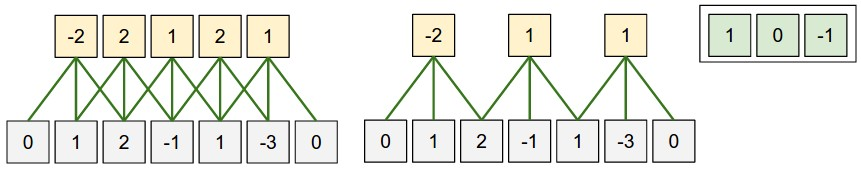weight=tf.get_variable(shape=[kernel_size, kernel_size, input_size, depth]）
bias = tf.get_variable(shape=[depth])
conv = tf.nn.conv2d(x, weight, strides=[1, 1, 1, 1], padding=‘SAME’)
conv_relu = tf.nn.relu(conv + bias)

### 池化 (Pooling)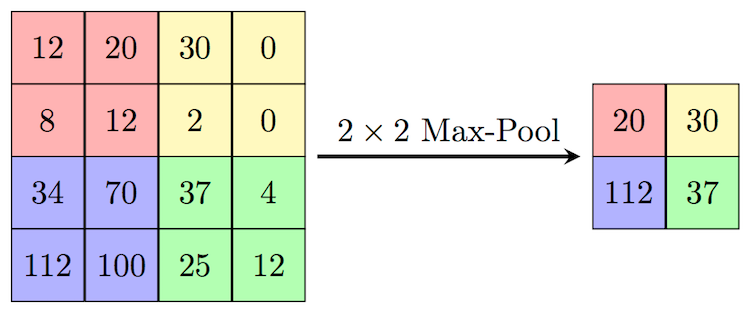tf.nn.max_pool(x, ksize=[1, size, size, 1], strides=[1, stride, stride, 1], padding=‘SAME’)

### 卷积神经网络的经典网络结构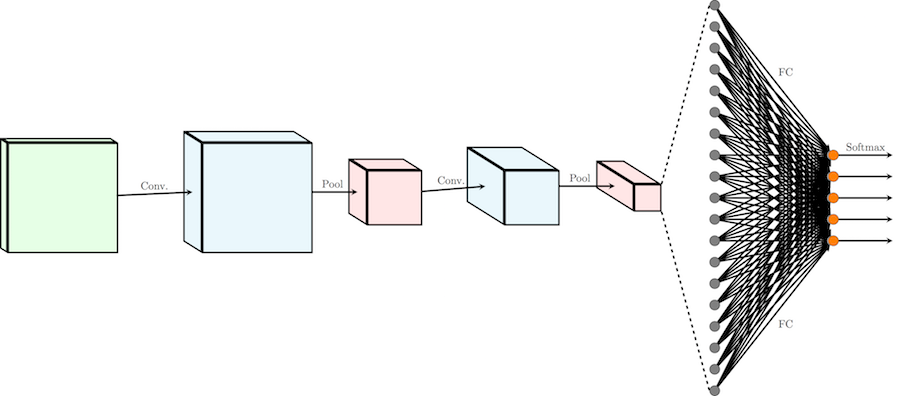• 一系列级联的 conv+pooling 层（有时会省略掉 pooling 层）。在级联的过程中，输入的尺寸逐渐变小，同时输出的 channel 逐渐变多，完成对信息从低级到高级的抽象。
• 一系列级联的全连接层。在卷积层到全连接层的交界处，卷积层的输出转化成一维的输入送入全连接层。之后根据任务的复杂程度，级联一系列全连接层。
• 最后的输出层，根据任务的需要，决定输出的形式。 如多分类问题，最后会接一个 softmax 层。

## 全卷积网络（Fully Convolution Network）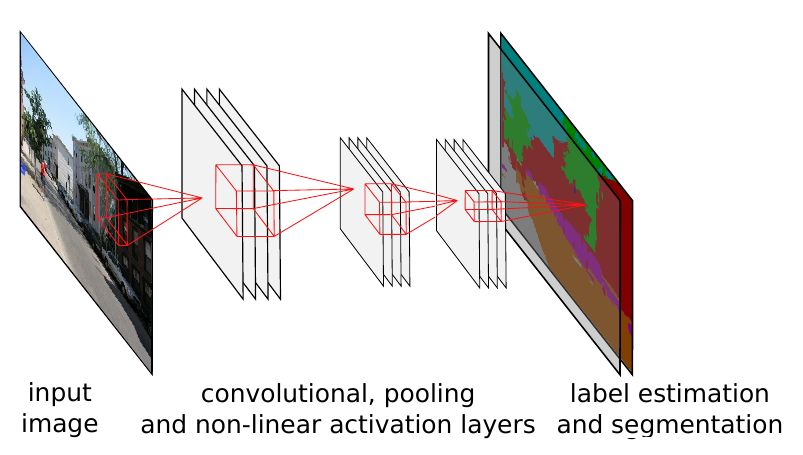### 反卷积（Deconvolution）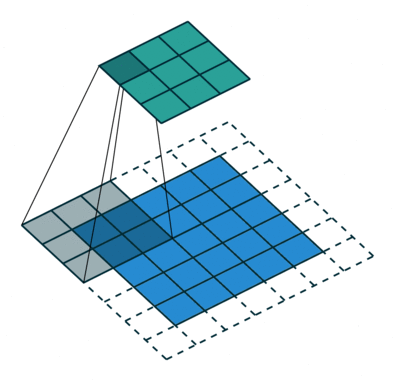## 卷积神经网络在视觉识别中的应用

CNN 在视觉识别（Visual Recognition）中有着非常广泛的应用。我们接下来以视觉识别中的三大经典问题：分类 / 回归、检测和分割为例，介绍如何用 CNN 解决实际问题。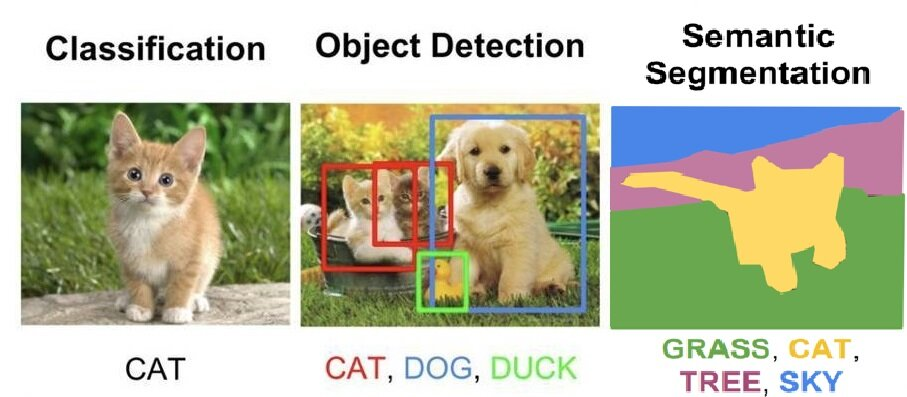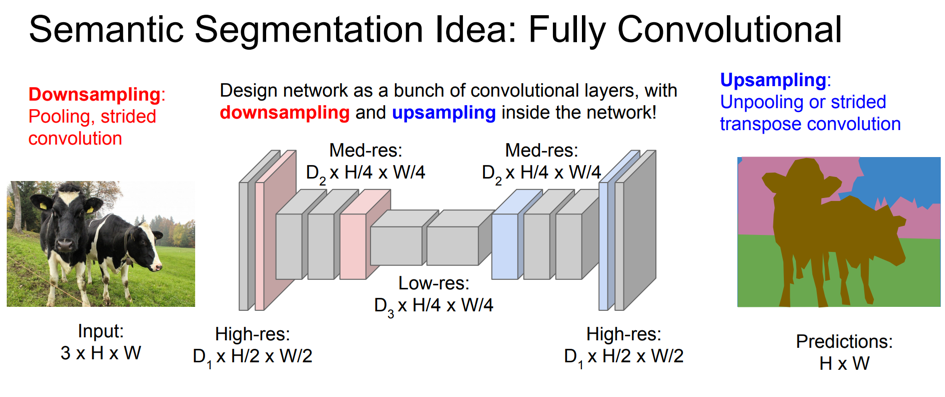## 实战： 人脸关键点检测### L2 距离回归

Kaggle 比赛的目标是预测人脸上 15 个关键点的坐标，总共 30 个 float 值，属于标准的回归问题。我们选择采用最常见的 l2 距离，作为优化的目标。和第一篇文章中神经网络模型的代码结构一样，我们将代码分成了 3 个主要模块，分别是 Dataset 模块，Net 模块和 Solver 模块。

• inference
我们在 inference 函数中定义网络的主体结构。因为模型会重复用到全连接层和卷积层，因此我们将他们封装成函数linear_reluconv_relu，从而方便复用代码。 网络结构上我们采用了比较简单的 3 层卷积，2 层全连接的结构。卷积层的输出通过tf.reshape转化成了全连接层可以接受的格式。因为是回归问题，我们直接将最后一层全连接层的结果作为输出。
• loss
为了简单，对于标准的回归问题，我们使用 mse 作为损失函数tf.reduce_mean(tf.square(predictions - labels), name='mse')
• metric
测试时，我们依旧使用 tensorflow 提供了 tf.metrics 模块，自动完成对每个 batch 的评价，并将所有的评价汇总。在这个例子里，我们是解决回归问题，因此可以使用tf.metrics.mean_squared_error计算均方误差。

def linear(x, output_size, wd=0):   input_size = x.get_shape().value  weight = tf.get_variable(      name='weight',      shape=[input_size, output_size],      initializer=tf.contrib.layers.xavier_initializer())  bias = tf.get_variable(      'bias', shape=[output_size], initializer=tf.constant_initializer(0.0))  out = tf.matmul(x, weight) + bias   if wd != 0:    weight_decay = tf.multiply(tf.nn.l2_loss(weight), wd, name='weight_loss')    tf.add_to_collection('losses', weight_decay)   return out  def linear_relu(x, output_size, wd=0):  return tf.nn.relu(      linear(x, output_size, wd), name=tf.get_default_graph().get_name_scope())  def conv_relu(x, kernel_size, width, wd=0):   input_size = x.get_shape()  weight = tf.get_variable(      name='weight',      shape=[kernel_size, kernel_size, input_size, width],      initializer=tf.contrib.layers.xavier_initializer())  bias = tf.get_variable(      'bias', shape=[width], initializer=tf.constant_initializer(0.0))  conv = tf.nn.conv2d(x, weight, strides=[1, 1, 1, 1], padding='SAME')   if wd != 0:    weight_decay = tf.multiply(tf.nn.l2_loss(weight), wd, name='weight_loss')    tf.add_to_collection('losses', weight_decay)   out = tf.nn.relu(conv + bias, name=tf.get_default_graph().get_name_scope())  return out  def pool(x, size):  return tf.nn.max_pool(      x, ksize=[1, size, size, 1], strides=[1, size, size, 1], padding='SAME')

class BasicCNN(Net):   def __init__(self, **kwargs):    self.output_size = kwargs.get('output_size', 1)    return   def inference(self, data):     with tf.variable_scope('conv1'):      conv1 = conv_relu(data, kernel_size=3, width=32)      pool1 = pool(conv1, size=2)     with tf.variable_scope('conv2'):      conv2 = conv_relu(pool1, kernel_size=2, width=64)      pool2 = pool(conv2, size=2)     with tf.variable_scope('conv3'):      conv3 = conv_relu(pool2, kernel_size=2, width=128)      pool3 = pool(conv3, size=2)     # Flatten convolutional layers output    shape = pool3.get_shape().as_list()    flattened = tf.reshape(pool3, [-1, shape * shape * shape])     # Fully connected layers    with tf.variable_scope('fc4'):      fc4 = linear_relu(flattened, output_size=100)     with tf.variable_scope('fc5'):      fc5 = linear_relu(fc4, output_size=100)     with tf.variable_scope('out'):      prediction = linear(fc5, output_size=self.output_size)     return {"predictions": prediction, 'data': data}   def loss(self, layers, labels):    predictions = layers['predictions']    with tf.variable_scope('losses'):      loss = tf.reduce_mean(tf.square(predictions - labels), name='mse')    return loss   def metric(self, layers, labels):    predictions = layers['predictions']    with tf.variable_scope('metrics'):      metrics = {        "mse": tf.metrics.mean_squared_error(          labels=labels, predictions=predictions)}    return metrics

Dataset

images = np.vstack(df['Image'].values) / 255.  # scale pixel values to [0, 1]images = images.astype(np.float32) label = df[df.columns[:-1]].valueslabel = (label - 48) / 48  # scale target coordinates to [-1, 1]label = label.astype(np.float32)

def parse_example(example_proto):  features = {      "data": tf.FixedLenFeature((9216), tf.float32),      "label": tf.FixedLenFeature((30), tf.float32, default_value=[0.0] * 30),  }  parsed_features = tf.parse_single_example(example_proto, features)  image = tf.reshape(parsed_features["data"], (96, 96, -1))  return image, parsed_features["label"]  dataset = tf.contrib.data.TFRecordDataset(files)dataset = dataset.map(self.parse_function)

Dataset 部分，我们使用了 tensorflow 推荐的 tfrecord 格式。通过TFRecordDataset函数读取 tfrecord 文件，并通过parse_example将 tfrecod 转换成模型的输入格式。tfrecord 作为一种定长格式，可以大大加快数据的读取速递。特别在使用 GPU 时，可以防止数据 io 成为性能的瓶颈。

Solver

file_dict = {    'train': os.path.join(args.data_dir, 'train.tfrecords'),    'eval': os.path.join(args.data_dir, 'test.tfrecords')} with tf.Graph().as_default():  dataset = Dataset(      file_dict=file_dict,      split='train',      parse_function=parse_example,      batch_size=50)  net = Net(output_size=30)  solver = Solver(dataset, net, max_steps=200, summary_iter=10)  solver.train()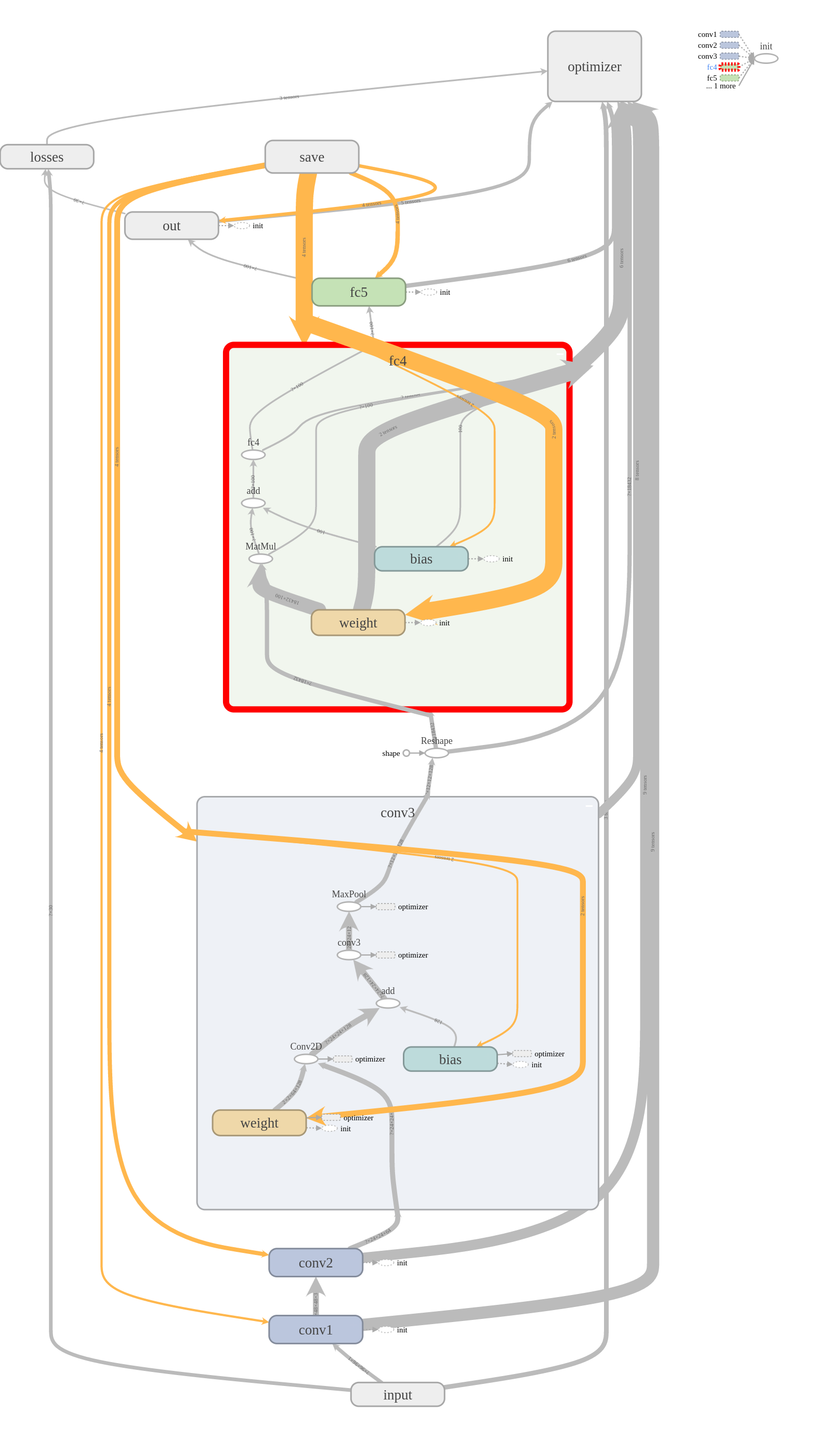step     10: loss = 0.0756 (136.2 examples/sec)step     20: loss = 0.0230 (155.2 examples/sec)step     30: loss = 0.0102 (149.1 examples/sec)step     40: loss = 0.0071 (125.1 examples/sec)step     50: loss = 0.0065 (160.9 examples/sec)step     60: loss = 0.0081 (171.9 examples/sec)step     70: loss = 0.0058 (148.4 examples/sec)step     80: loss = 0.0060 (169.4 examples/sec)step     90: loss = 0.0069 (185.4 examples/sec)step    100: loss = 0.0057 (186.1 examples/sec)step    110: loss = 0.0062 (183.8 examples/sec)step    120: loss = 0.0080 (170.3 examples/sec)step    130: loss = 0.0052 (185.8 examples/sec)step    140: loss = 0.0071 (184.3 examples/sec)step    150: loss = 0.0049 (170.7 examples/sec)step    160: loss = 0.0056 (178.7 examples/sec)step    170: loss = 0.0053 (173.2 examples/sec)step    180: loss = 0.0058 (172.6 examples/sec)step    190: loss = 0.0053 (172.5 examples/sec)step    200: loss = 0.0056 (188.1 examples/sec) mse: 0.140243709087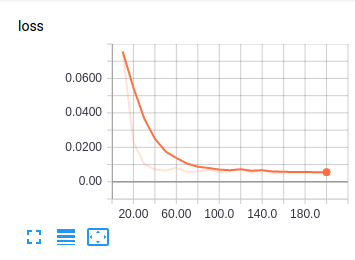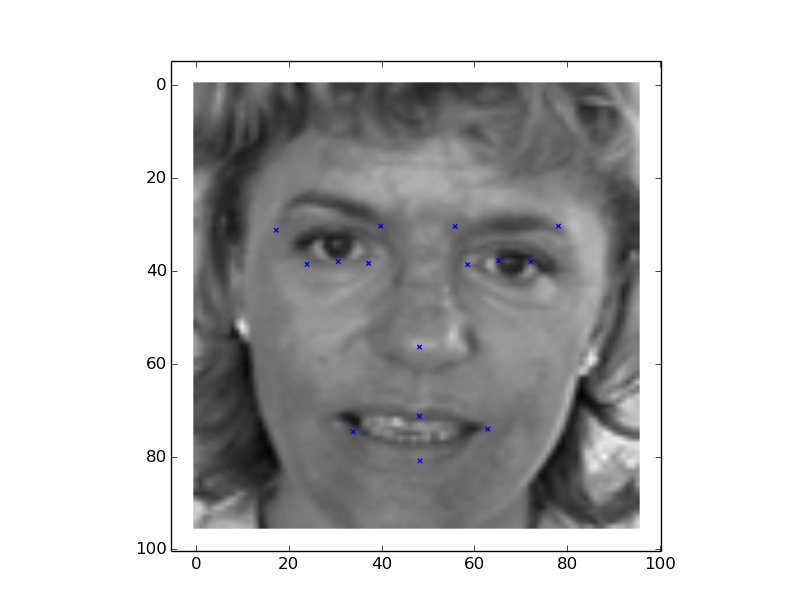2017 年 12 月 14 日 16:537505

## 评论

• ###### 带你跨过神经网络训练常见的 37 个坑

近日，Slav Ivanov发表了一篇题为《37 reasons why your neural network is not working》的文章，从数据集、数据归一化/增强、实现以及训练四个方面，总结了37条自己对神经网络的调试经验，希望能帮助读者解决神经网络训练中经常遇到的问题。

• ###### MXNet API 入门 —第 4 篇

Apache MXNet是一种功能全面、可以灵活编程并且扩展能力超强的深度学习框架，支持包括卷积神经网络(CNN)与长短期记忆网络(LSTM)在内的顶尖深度模型。这一系列文章介绍了MXNet的基本概念和使用方法。本篇主要介绍如何使用训练模型进行图片分类。

• ###### 17 人工神经网络 | 一个青年才俊的意外死亡：神经元与感知器

1943年，一篇论文宣告了人工神经网络的呱呱坠地，神经网络的鼻祖感知器的基本原理是怎样的？

2018 年 1 月 16 日

• ###### 如何用向量和参数方程描述曲线？

不论我们用什么图形系统绘图，图形的呈现都离不开曲线。那我们该如何描述曲线呢？

2020 年 7 月 6 日

• ###### Embedding+MLP：如何用 TensorFlow 实现经典的深度学习模型？

你知道微软在2016年提出的深度学习模型Deep Crossing吗？它就属于经典的Embedding+MLP模型。

2020 年 11 月 18 日

• ###### Hinton 领衔谷歌大脑新研究，通过胶囊网络重构自动检测对抗样本

胶囊网络（capsule network，CapsNet）可以自动把对抗样本揪出来啦！这项研究来自 capsule 模型的原作者 Sabour、Frosst 以及 Hinton，相关论文将在 NIPS 2018 的机器学习安全研讨会中展示。

• ###### 基于深度学习的图像分割在高德的实践

本文为《高德技术2019年刊合辑》系列之一。

• ###### 简述无监督图像分类发展现状

无监督图像分类问题是图像分类领域一项极具挑战的研究课题，本文介绍了无监督图像分类算法的发展现状，供参考学习。

• ###### 腾讯冯牮：基于卷积神经网络在手机端实现文档检测

2017年10月17日至19日，有InfoQ举办的全球软件开发大会QCon将在上海举办。大会吸引了来自世界各地的技术专家及开发者。本文采访了QCon大会的参会讲师，来自腾讯的的iOS高级开发工程师冯牮，本次大会他的演讲题目为《基于卷积神经网络在手机端实现文档检测》。

• ###### 如何用仿射变换来移动和旋转 3D 物体？

在三维世界中，想要移动和旋转物体，也需要使用仿射变换。那仿射变换该怎么从二维扩展到三维几何空间呢？

2020 年 8 月 12 日

• ###### 深入浅出 Tensorflow（四）：卷积神经网络

本文将介绍卷积神经网络，通过与传统算法的对比、卷积神经网络的结构分析等方面来介绍卷积神经网络模型，并给出通过TensorFlow在MNIST数据集上实现卷积神经网络的方法。

• ###### 深入浅出 Tensorflow（五）：循环神经网络简介

本文主要介绍循环神经网络。该网络结构源于霍普菲尔德网络，在语义信息深度表达表达、语音识别、语言建模、机器翻译和时序分析等方面实现了突破。本文将以几个典型的循环神经网络结构为例进行讲解，并给出实验代码加以说明，并附以一个Tensorflow样例来使用循环神经网络实现语言模型。

• ###### 25 丨 KNN（下）：如何对手写数字进行识别？

今天我用KNN、SVM、朴素贝叶斯和决策树做分类器，分别做一下手写数字分类识别的实战。

2019 年 2 月 8 日

• ###### 运用计算图搭建卷积神经网络

本文介绍如何运用计算图搭建卷积神经网络。

• ###### 机器学习入门之 HelloWorld（下）

Wx+b，这是线性关系。在很多问题中其解法并非线性关系能完成的，在深度学习，能过能多层卷积神经网络组合非线性激活函数来模拟更复杂的非线性关系，效果往往比单一的线性关系更好。

• ###### 43 丨深度学习（下）：如何用 Keras 搭建深度学习网络做手写数字识别？

我们之前用KNN算过手写数字识别这个项目，这节课我们用Keras这个深度学习框架做一下，两者之间到底有什么区别呢？

2019 年 3 月 22 日

• ###### 用 Python 从零开始构建 ResNet

这些年来，深度学习架构变得越来越深（层越来越多）以解决越来越复杂的任务，这也有助于提高分类和识别任务的性能，并让它们表现稳健。但当我们继续向神经网络添加更多层时，模型训练起来也越来越困难，模型的准确度开始饱和，然后还会下降。于是ResNet诞生了，让我们摆脱了这种窘境，并能帮助解决这个问题。

• ###### 虹膜识别技术

本文来自微信京东数科技术说公众号。

• ###### 深度学习时代的目标检测算法综述

计算机视觉领域从来就不乏有意思的课题，从单张图片的分类，到3D姿态估计。其中我们最感兴趣的，以及投入大量精力的课题之一就是目标检测。就像其他许多计算机视觉问题，目前仍然缺乏一个显而易见的或“最好的”方法来解决这一问题，这也意味着改进的空间还很大。在进入目标检测领域之前，让我们快速的过一遍该领域最常见的问题。

#### 硬核！阿里内部这份《Java面试核心知识手册》在Github上已获赞高达89.7K！